# Mathematics - Signals And Systems - Continuous-Time Modulation[Image1]

## Introduction

Hey it's a me again @drifter1!

Today we continue with my mathematics series about Signals and Systems in order to cover Continuous-Time Modulation.

So, without further ado, let's dive straight into it!

## Modulation Property

Let's first recall what modulation means in the context of Signals and Systems. Modulation is one of the various useful properties of the Fourier Transform, and is basically the FT of the multiplication of two signals. As we know, the product/multiplication of two signals turns into a convolution of their individual Foruier Transforms.

Consider x(t) to be the continuous-time signal to be "modulated". The signal c(t) which is used to modulate x(t) is known as the carrier signal. Various types of carriers can be used, which we will get into in a bit. As a result, the modulation property can be written as:## Getting into Modulation

Modulation is a very important concept used in communication systems, and also the basis for converting between continuous-time and discrete-time signals. To put things simply, think of the information being embedded into the carrier signal by tweaking/modulating some parameter of it.

The two basic modulations are amplitude and frequency modulation. In amplitude modulation, the information to be transmitted modulates the amplitude of the carrier, whilst the carrier signal that transmits it is a sinusoidal signal of specific frequency. On the other hand, in frequency modulation, the information modulates the frequency of the carrier, transmitting the information in the amplitude of the carrier signal.

So, remember the following:

• Amplitude Modulation (AM) = Transmission in the Frequency of the Carrier Signal
• Frequency Modulation (FM) = Transmission in the Amplitude of the Carrier Signal

## Amplitude Modulation

Let's start of with amplitude modulation. Graphically, AM looks as follows:
[Image 2]

AM is defined by the simple modulation/property that we covered before, and so the outcome is as follows:The carrier signal can be a:

• pulse signal
• sinusoidal signal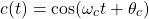• complex exponential signal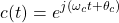For example, using a sinusodial carrier yields: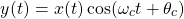## Frequency Modulation

Next up is FM, which graphically does the following: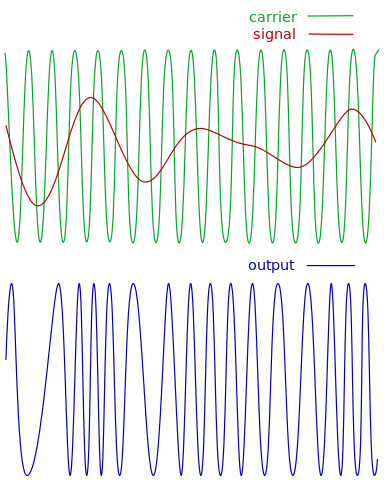[Image 3]

In FM, the frequency of the carrier is modulated, which in turns means that the the input signal modulates the phase of the carrier signal in some manner. Using a sinusoidal carrier, its easy to define FM as: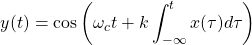Tweaking the parameter k allows us to widen/narrow the bandwidth of the transmission.

If we applied x(t) directly, the outcome would be Phase Modulation (PM):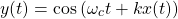## Demodulation

In addition to modulation there is also the concept of demodulation. Using a complex exponential carrier the original signal can be recovered very easily, by modulating a second time using the complex conjugate signal.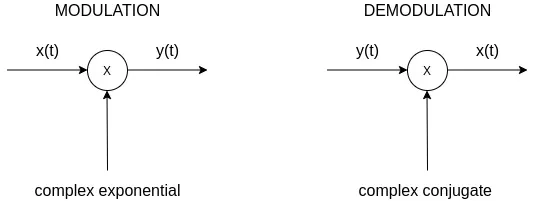Contrarily, the recovery of the signal using a sinusoidal carrier is a two step procedure. Demodulation consists of modulating again with a sinusodial carrier, followed by low-pass filtering that "extracts" the original signal.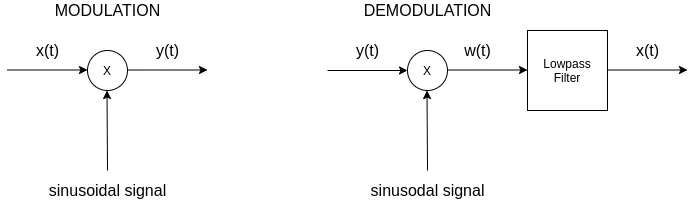Because synchronization is quite difficult to achieve, demodulation is easier to implement asynchronously. But, such a less expensive demodulator suffers of inefficiency in power transmission.

## RESOURCES:

### Images

1.2.3.Block diagrams and other visualizations were made using draw.io and GeoGebra

## Previous articles of the series

### Filtering, Sampling, Modulation, Interpolation

• Filtering → Convolution Property, Ideal Filters, Series R-C Circuit and Moving Average Filter Approximations

## Final words | Next up

And this is actually it for today's post!

Next time we will get into Discrete-Time Modulation...

See Ya!Keep on drifting!

H2
H3
H4
3 columns
2 columns
1 column
Join the conversation now